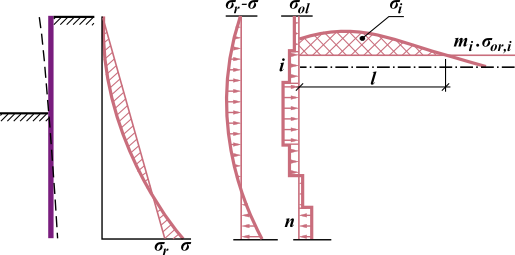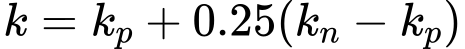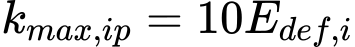O link foi enviado para o seu email.

Não foi possível enviar o link para o seu email. Por favor, verifique o seu email.

# Ajuda Online

Tree
Settings
Produto:
Programa:
Idioma:

## Modulus of Subsoil Reaction Determined from Iteration

The program allows automatic calculation of the modulus of subsoil reaction from deformational characteristics of soil from iteration process. The procedure builds on the assumption that deformation of the elastic subspace characterized by the deformation modulus Edef [MPa] when changing the stress state associated with the change of earth pressures is the same as deformation of the underground wall.

The goal therefore is to find such values of kh [MN/m3] so that the continuity of deformations of wall and adjacent soil is maintained. Plastic deformation of structure is not considered when performing analysis with kh manual iteration. While the analysis of modulus kh with automatic iteration plastic deformation of structure is considered. Principle of manual iteration is schematically cleared by computing the modulus of subsoil reaction of the ith segment of wall free of anchor, see figure:Determination of modulus of subsoil reaction of ith segment

For change of stress σr - σ the program determines uniform load σol [MPa] of individual segments of a structure. Next, the overall change of stress passing the ith segment ([MPa*m]) is computed. This change is caused by additional load of the soil body due to segments 1 to n (σol,1 - σol,n). The overall change of stress Δσi is reduced by structural strength mi*σor,i [MPa]. The new value of the spring stiffness then follows from:where: Edef - deformation modulus of elastic subspace [MPa] σol - uniform load applied to segments of structure [MPa]- overall change of stress behind ith segment of structure [MPa*m]

The change of stress inside the soil body is determined according to Boussinesque. Inserting the new value of k directly into the next calculation would cause instable iteration - therefore the value of k that is introduced into the next analysis of the wall is determined from the original value of kp and the new value of kn of the modulus of subsoil reaction.where: kp - original value of modulus of subsoil reaction [MN/m3] kn - new value of modulus of subsoil reaction [MN/m3]

Maximum of modulus of subsoil reaction of the ith layer is limited by the value:where: Edef,i - deformation modulus of ith layer [MPa]

The manual iterative procedure used when computing the modulus of subsoil reaction is as follows:

1. Determine the matrix of influence values for deriving change of stress in a depth of the soil body passing the ith segment of a structure due to surcharge caused by the change of stress in other segments.
2. The first approximation of the modulus kh in front of the wall is introduced - a triangular distribution of values at the wall heel kh = 10 MN/m3 is assumed.
3. Perform analysis of the wall (sheeting structure).
4. Compute new values of kh and determine new values for the next analysis.
5. The dialog window to check the iteration appears and the program waits till the next command. If the next n iterations are selected, the steps 3 and 4 are repeated n-times to arrive again at the step No. 5. The analysis is terminated in this dialog window by pressing the "Stop" button.

This manual iterative process is controlled by the user - he or she has to decide whether the results make sense. Automatic iterative procedure is performed without entering of further iterations for calculation of modulus kh.

Literature:

Bartak J.: Progresivni postupy navrhovani pazenych stavebnich jam, VUT Brno, 1991.